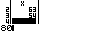Casio Calculator Tutorials

#Mean, Sum & Count – fx-83GTX

Calculating the mean or sum of a set of data, or even counting the set of data for use in other formula is easy with Stat mode, available on both types of calculator.

For example, I will calculate the mean and sum of the following set:

1. Enter STAT mode by pressing MODE 2.
2. Select 1-VAR by pressing 1.
3. Enter the values in the set of data, using = to move down to the next cell.
Use ↑ and ↓ to move up and down to correct inaccuracies.
4. Press AC to exit the data.

The sum of the data is shown using
SHIFT 1 4 (Sum) 2 (∑x).

The mean of the data is shown using
SHIFT 1 5 (Var) 2 (X¯).

The number of values n is shown using
SHIFT 1 5 (Var) 1 (n).

Buy a Casio fx-83GTX calculator on Amazon using this affiliate link to help support this site.

00